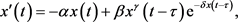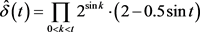﻿ 时滞脉冲新古典增长模型的正周期解 Positive Periodic Solutions for a Class of Delayed Neoclassical Growth Model

Vol.06 No.06(2017), Article ID:22107,7 pages
10.12677/AAM.2017.66087

Positive Periodic Solutions for a Class of Delayed Neoclassical Growth Model

Chunyu Yang, Ruojun Zhang, Jingjing Zhang

School of Mathematical Sciences, Ocean University of China, Qingdao ShandongReceived: Aug. 25th, 2017; accepted: Sep. 11th, 2017; published: Sep. 19th, 2017ABSTRACT

In this paper, the existence of positive periodic solutions for a class of delayed neoclassical growth model with impulse is considered. By using the cone fixed point theorem, some sufficient conditions of the existence of positive periodic solutions for the addressed model are obtained. Moreover, an example is given to show the effectiveness of our results.

Keywords:Neoclassical Growth Model, Positive Periodic Solutions, Delay, Impulse1. 引言

20世纪50年代，索洛等人   提出形如 $Y\left(t\right)=A\left(t\right)F\left(K\left(t\right),L\left(t\right)\right)$ 的新古典增长模型，其中 $Y\left(t\right)$时期的总产出， $K\left(t\right)$$t$ 时期投入的资本量， $L\left(t\right)$$t$ 时期投入的劳动量， $A\left(t\right)$ 代表 $t$ 时期的技术水平，由于具有预见性与实用性，该模型被众多学者所关注。Day    ，Puu  ，Bischi  等人进一步研究了新古典增长模型等非线性经济动力系统，并对之有极大的创新和发展。依据经济学原理，为描述长时间经济行为，新古典增长模型有两个基本假设：一是劳动力和资本充分；二是输出市场的即时调整。然而，由于生产过程中时滞的不可避免，所以这种理想的假设在现实中是不合理的，因此有必要考虑时滞系统。

2011年，Matsumoto和Szidarovszky  首次介绍了如下的时滞新古典增长模型

${x}^{\prime }\left(t\right)=-\alpha x\left(t\right)+sF\left(x\left(t-\tau \right)\right),$ (1.1)(1.2)

2. 预备知识

$\left\{\begin{array}{l}{x}^{\prime }\left(t\right)=-\alpha \left(t\right)x\left(t\right)+\beta \left(t\right){x}^{\gamma }\left(t-\tau \left(t\right)\right){\text{e}}^{-\delta \left(t\right)x\left(t-\tau \left(t\right)\right)}\text{\hspace{0.17em}}\text{\hspace{0.17em}}t\ne {t}_{k},t\ge {t}_{0}>0\\ \Delta x\left({t}_{k}\right)=x\left({t}_{k}^{+}\right)-x\left({t}_{k}^{-}\right)={p}_{k}x\left({t}_{k}\right)\text{\hspace{0.17em}}\text{ }\text{ }\text{ }\text{ }\text{ }\text{ }\text{\hspace{0.17em}}\text{ }k=1,2\cdots \end{array}$ (2.1)

$x\left(t\right)=\varphi \left(t\right),\text{\hspace{0.17em}}\text{\hspace{0.17em}}t\in \left[-\tau ,0\right]。$ (2.2)

H1) $\alpha \left(t\right),\beta \left(t\right),\delta \left(t\right),\tau \left(t\right)$ 是以 $\omega$ 为周期的周期函数， $\omega >0$

H2) ${t}_{0}<{t}_{1}<{t}_{2}<\cdots$${t}_{i},\text{\hspace{0.17em}}i=1,2,\cdot \cdot \cdot$ 为给定的脉冲时刻， $\underset{k\to \infty }{\mathrm{lim}}{t}_{k}=+\infty$

H3) $\left\{{p}_{k}\right\}$ 为实数列， ${p}_{k}\ne -1,k=1,2,\cdots$

H4) $\underset{{t}_{0}<{t}_{k} 为以 $\omega$ 为周期的周期函数(这里作一个标准的假设，若因子个数为0，则乘积为1)；

H5) ${\int }_{0}^{\omega }\alpha \left(t\right)\text{d}t>0$

i) $x\left(t\right)$$\left({t}_{0},{t}_{1}\right]$$\left({t}_{k},{t}_{k+1}\right],k=1,2,\cdots$ 上绝对连续；

ii) $\forall {t}_{k},k=1,2,\cdots ,x\left({t}_{k}^{+}\right)$$x\left({t}_{k}^{-}\right)$ 存在且 $x\left({t}_{k}^{-}\right)=x\left({t}_{k}\right)$

iii) $x\left(t\right)$$\left[{t}_{0},+\infty \right)\{t}_{k}$ 上几乎处处满足方程(2.1)，在 $t={t}_{k},k=1,2,\cdots$ 满足脉冲条件；

iv) $x\left(t\right)=\varphi \left(t\right),t\in \left[-\tau ,0\right]$

${y}^{\prime }\left(t\right)=-\alpha \left(t\right)x\left(t\right)+\stackrel{^}{\beta }\left(t\right){y}^{\gamma }\left(t-\tau \left(t\right)\right){\text{e}}^{-\stackrel{^}{\delta }\left(t\right)y\left(t-\tau \left(t\right)\right)},$ (2.3)

$y\left(t\right)=\varphi \left(t\right),\text{\hspace{0.17em}}t\in \left[-\tau ,0\right]。$ (2.4)

$y\left(t\right)$ 是模型(2.3)在初值条件(2.4)下的解，是指 $y\left(t\right)$ 是定义在 $\left[{t}_{0}-\tau ,+\infty \right)$ 上，在 $\left[{t}_{0},+\infty \right)$ 上是满足(2.3)的绝对连续函数，且在 $\left[{t}_{0}-\tau ,{t}_{0}\right]$ 上满足初值条件(2.4)。

i) $ax+by\in P,\text{\hspace{0.17em}}\text{\hspace{0.17em}}\forall x,y\in P,\text{\hspace{0.17em}}a,b>0。$

ii) $x,-x\in P$ 蕴含 $x=0$

${\Omega }_{1},{\Omega }_{2}$ 为Banach空间 $X$ 中的有界开子集， $\theta \in {\Omega }_{1},{\stackrel{¯}{\Omega }}_{1}\subset {\Omega }_{2}$$P$$X$ 中的一个锥， $T:P\cap \left({\stackrel{¯}{\Omega }}_{2}\{\Omega }_{1}\right)\to P$ 为全连续算子，若 $T$ 满足条件：

i) $‖Tx‖\le ‖x‖,\forall x\in P\cap \partial {\Omega }_{1};‖Tx‖\ge ‖x‖,\forall x\in P\cap \partial {\Omega }_{2}$ (即范数锥拉伸)；

ii) $‖Tx‖\le ‖x‖,\forall x\in P\cap \partial {\Omega }_{2};‖Tx‖\ge ‖x‖,\forall x\in P\cap \partial {\Omega }_{1}$ (即范数锥压缩)。

$T$$P\cap \left({\stackrel{¯}{\Omega }}_{2}\{\Omega }_{1}\right)$ 中必存在不动点。

3. 主要结果

i) $y\left(t\right)$ 为模型(2.3)与(2.4)的解，则  为模型(2.1)与(2.2)在 $\left[{t}_{0}-\tau ,+\infty \right)$ 上的解；

ii) $x\left(t\right)$ 为模型(2.1)与(2.2)的解，则 $y\left(t\right)=\underset{{t}_{0}<{t}_{k} 为模型(2.3)与(2.4)在 $\left[{t}_{0}-\tau ,+\infty \right)$ 上的解。

$\begin{array}{l}{x}^{\prime }\left(t\right)={\left[\underset{{t}_{0}<{t}_{k}0。\end{array}$

$x\left({t}_{k}^{+}\right)=\underset{t\to {t}_{k}^{+}}{\mathrm{lim}}\underset{{t}_{0}<{t}_{j}

ii)设 $x\left(t\right)$ 是模型(2.1)与(2.2)的解，所以 $x\left(t\right)$$\left({t}_{0},{t}_{1}\right]$$\left({t}_{k},{t}_{k+1}\right],k=1,2,\cdots$ 上是绝对连续的。因此， $y\left(t\right)=\underset{{t}_{0}<{t}_{k}$\left({t}_{0},{t}_{1}\right]$$\left({t}_{k},{t}_{k+1}\right],k=1,2,\cdots$ 上也是绝对连续的。

$\forall t={t}_{k},k=1,2,\cdots$ ，有

$y\left({t}_{k}^{+}\right)=\underset{t\to {t}_{k}^{+}}{\mathrm{lim}}\underset{{t}_{0}<{t}_{j}

$y\left({t}_{k}^{-1}\right)=\underset{t\to {t}_{k}^{-1}}{\mathrm{lim}}\underset{{t}_{0}<{t}_{j} ，则 $y\left(t\right)$ 是连续的且易知在 $\left[{t}_{0},+\infty \right)$ 上绝对连续，由(i)类似可证 $y\left(t\right)$ 为(2.3)在 $\left[{t}_{0}-\tau ,+\infty \right)$ 上满足初值条件(2.4)的解。

i) $y\left(t\right)$ 为(2.3)与(2.4)的 $\omega$ -周期解，则 $x\left(t\right)=\underset{{t}_{0}<{t}_{k} 为(2.1)与(2.2)在 $\left[{t}_{0}-\tau ,+\infty \right)$ 上的正 $\omega$ -周期解；

ii) $x\left(t\right)$ 为(2.1)与(2.2)的 $\omega$ -周期解，则 $y\left(t\right)=\underset{{t}_{0}<{t}_{k} 为(2.3)与(2.4)在 $\left[{t}_{0}-\tau ,+\infty \right)$ 上的正 $\omega$ -周期解。

$P=\left\{x\left(t\right)\in X|x\left(t\right)\ge 0,x\left(t\right)\ge \sigma ‖x‖,t\in \text{R}\right\}$ ，其中 $\sigma \in \left(0,1\right)$ 为后面所定义的正常数，则 $P$$X$ 中的锥。定义算子

$\left(\Gamma x\right)\left(t\right)={\int }_{t}^{t+\omega }G\left(t,s\right)\stackrel{^}{\beta }\left(s\right){x}^{\gamma }\left(s-\tau \left(s\right)\right){\text{e}}^{-\stackrel{^}{\delta }\left(s\right)x\left(s-\tau \left(s\right)\right)}\text{d}s$ (3.1)

$0

$G\left(t+\omega ,s+\omega \right)=G\left(t,s\right),s\in \left[t,t+\omega \right]$

$\forall x\in P,t\in \text{R}$ ，有

$\begin{array}{l}\left(\Gamma x\right)\left(t+\omega \right)={\int }_{t+\omega }^{t+2\omega }G\left(t+\omega ,s\right)\stackrel{^}{\beta }\left(s\right){x}^{\gamma }\left(s-\tau \left(s\right)\right){\text{e}}^{-\stackrel{^}{\delta }\left(s\right)x\left(s-\tau \left(s\right)\right)}\text{d}s\\ \text{ }\text{ }\text{ }\text{ }\text{\hspace{0.17em}}\text{\hspace{0.17em}}\stackrel{令s=u+\omega }{=}{\int }_{t}^{t+\omega }G\left(t+\omega ,u+\omega \right)\stackrel{^}{\beta }\left(u+\omega \right){x}^{\gamma }\left(u+\omega -\tau \right){\text{e}}^{-\stackrel{^}{\delta }\left(u+\omega \right)x\left(u+\omega -\tau \left(u+\omega \right)\right)}\text{d}u\\ \text{ }\text{ }\text{ }\text{ }\text{\hspace{0.17em}}\text{\hspace{0.17em}}={\int }_{t}^{t+\omega }G\left(t,u\right)\stackrel{^}{\beta }\left(u\right){x}^{\gamma }\left(u-\tau \left(u\right)\right){\text{e}}^{-\stackrel{^}{\delta }\left(u\right)x\left(u-\tau \left(u\right)\right)}\text{d}u\\ \text{ }\text{ }\text{ }\text{ }\text{\hspace{0.17em}}\text{\hspace{0.17em}}=\left(\Gamma x\right)\left(t\right),\end{array}$

$\left(\Gamma x\right)\left(t\right)\ge A{\int }_{0}^{\omega }\stackrel{^}{\beta }\left(s\right){x}^{\gamma }\left(s-\tau \left(s\right)\right){\text{e}}^{-\stackrel{^}{\delta }\left(s\right)x\left(s-\tau \left(s\right)\right)}\text{d}s\ge \frac{A}{B}‖\Gamma x‖=\sigma ‖\Gamma x‖$

$\left(\Gamma x\right)\left(t\right)\le B{\int }_{0}^{\omega }\stackrel{^}{\beta }\left(s\right){x}^{\gamma }\left(s-\tau \left(s\right)\right){\text{e}}^{-\stackrel{^}{\delta }\left(s\right)x\left(s-\tau \left(s\right)\right)}\text{d}s\le B\omega {\stackrel{^}{\beta }}_{M}g\left(\frac{\gamma }{{\stackrel{^}{\delta }}_{M}}\right)\le B\omega {\stackrel{^}{\beta }}_{M}{\text{e}}^{-1}{\left(\frac{\gamma }{{\stackrel{^}{\delta }}_{M}}\right)}^{\gamma }$

$\begin{array}{c}{\left(\Gamma x\right)}^{\prime }\left(t\right)={\left[{\int }_{t}^{t+\omega }G\left(t,s\right)\stackrel{^}{\beta }\left(s\right){x}^{\gamma }\left(s-\tau \left(s\right)\right){\text{e}}^{-\stackrel{^}{\delta }\left(s\right)x\left(s-\tau \left(s\right)\right)}\text{d}s\right]}^{\prime }\\ ={\int }_{t}^{t+\omega }{G}^{\prime }\left(t,s\right)\stackrel{^}{\beta }\left(s\right){x}^{\gamma }\left(s-\tau \left(s\right)\right){\text{e}}^{-\stackrel{^}{\delta }\left(s\right)x\left(s-\tau \left(s\right)\right)}\text{d}s\\ \text{\hspace{0.17em}}\text{\hspace{0.17em}}+G\left(t,t+\omega \right)\stackrel{^}{\beta }\left(t+\omega \right){x}^{\gamma }\left(t+\omega -\tau \left(t+\omega \right)\right){\text{e}}^{-\stackrel{^}{\delta }\left(t+\omega \right)x\left(t+\omega -\tau \left(t+\omega \right)\right)}\\ \text{\hspace{0.17em}}\text{\hspace{0.17em}}-G\left(t,t\right)\stackrel{^}{\beta }\left(t\right){x}^{\gamma }\left(t-\tau \left(t\right)\right){\text{e}}^{-\stackrel{^}{\delta }\left(t\right)x\left(t-\tau \left(t\right)\right)}\\ ={\int }_{t}^{t+\omega }{G}^{\prime }\left(t,s\right)\stackrel{^}{\beta }\left(s\right){x}^{\gamma }\left(s-\tau \left(s\right)\right){\text{e}}^{-\stackrel{^}{\delta }\left(s\right)x\left(s-\tau \left(s\right)\right)}\text{d}s\\ \le \alpha \left(t\right)\left(\Gamma x\right)\left(t\right)+\stackrel{^}{\beta }\left(t\right){\text{e}}^{-\stackrel{^}{\delta }\left(s\right)x\left(s-\tau \left(s\right)\right)}\\ \le {\alpha }_{M}B\omega {\stackrel{^}{\beta }}_{M}{\text{e}}^{-\text{1}}{\left(\frac{\gamma }{{\delta }_{M}}\right)}^{\gamma }+{\stackrel{^}{\beta }}_{M}{\text{e}}^{-{\stackrel{^}{\delta }}_{M}},\end{array}$

$\stackrel{^}{\beta }\left(t\right){x}^{\gamma }{\text{e}}^{-\stackrel{^}{\delta }\left(t\right)x}\le \epsilon {r}_{1},\text{\hspace{0.17em}}t\in \left[0,\omega \right],\text{\hspace{0.17em}}x\in \left[0,{r}_{1}\right];$ (3.2)

$\stackrel{^}{\beta }\left(t\right){x}^{\gamma }{\text{e}}^{-\stackrel{^}{\delta }\left(t\right)x}\le \epsilon {r}_{2},\text{\hspace{0.17em}}t\in \left[0,\omega \right],\text{\hspace{0.17em}}x\in \left[{r}_{2},+\infty \right)。$ (3.3)

$\left(\Gamma x\right)\left(t\right)\le B{\int }_{t}^{t+\omega }\stackrel{^}{\beta }\left(s\right){x}^{\gamma }\left(s-\tau \left(s\right)\right){\text{e}}^{-\stackrel{^}{\delta }\left(s\right)x\left(s-\tau \left(s\right)\right)}\text{d}s\le B\omega \epsilon {r}_{1}<{r}_{1}$

$\left(\Gamma x\right)\left(t\right)\ge A{\int }_{t}^{t+\omega }\stackrel{^}{\beta }\left(s\right){x}^{\gamma }\left(s-\tau \left(s\right)\right){\text{e}}^{-\stackrel{^}{\delta }\left(s\right)x\left(s-\tau \left(s\right)\right)}\text{d}s\ge A\omega {\stackrel{^}{\beta }}_{m}{r}_{0}{}^{\gamma }{\text{e}}^{-{\stackrel{^}{\delta }}_{M}{r}_{0}}>\frac{{r}_{0}}{{\text{e}}^{-{\stackrel{^}{\delta }}_{M}{r}_{0}}}{\text{e}}^{-{\stackrel{^}{\delta }}_{M}{r}_{0}}={r}_{0},$

$M=\underset{t\in \left[0,\omega \right]}{\mathrm{max}}\underset{x\in \left[0,{r}_{2}\right]}{\mathrm{max}}\left\{\stackrel{^}{\beta }\left(t\right){x}^{\gamma }{\text{e}}^{-\stackrel{^}{\delta }\left(t\right)x}\right\}$ ，当 $x\in P\cap \partial {\Omega }_{3}$ 时，有 $‖x‖={r}_{2}$$x\left(t\right)\ge \sigma {r}_{2}$ 。则由(3.1)和(3.3)，得

$\begin{array}{c}\left(\Gamma x\right)\left(t\right)\le B{\int }_{t}^{t+\omega }\stackrel{^}{\beta }\left(s\right){x}^{\gamma }\left(s-\tau \left(s\right)\right){\text{e}}^{-\stackrel{^}{\delta }\left(s\right)x\left(s-\tau \left(s\right)\right)}\text{d}s\\ \le B\left({\int }_{{E}_{1}}^{}\stackrel{^}{\beta }\left(s\right){x}^{\gamma }\left(s-\tau \left(s\right)\right){\text{e}}^{-\stackrel{^}{\delta }\left(s\right)x\left(s-\tau \left(s\right)\right)}\text{d}s+{\int }_{{E}_{2}}^{}\stackrel{^}{\beta }\left(s\right){x}^{\gamma }\left(s-\tau \left(s\right)\right){\text{e}}^{-\stackrel{^}{\delta }\left(s\right)x\left(s-\tau \left(s\right)\right)}\text{d}s\right)\\ \le BM\omega +B\omega \epsilon {r}_{2}<{r}_{2},\end{array}$

4. 具体实例

$\left\{\begin{array}{l}{x}^{\prime }\left(t\right)=-\left(0.\text{06}+\mathrm{sin}t\right)x\left(t\right)+\left(\text{7}+\mathrm{cos}t\right){x}^{\text{3}}\left(t-1\right){\text{e}}^{-\left(\text{2}-0.5\mathrm{sin}t\right)x\left(t-1\right)},\text{ }t\ne {t}_{k},\\ \Delta x\left(k\right)=\left(1+{p}_{k}\right)x\left(k\right),\text{ }\text{ }k=1,2,\cdots \end{array}$ (4.1)

$f\left(t+2\text{π}\right)=\underset{0<{t}_{k}<2\text{π}}{\prod }{2}^{\mathrm{sin}k}\cdot \underset{2\text{π}<{t}_{k}<2\text{π}+t}{\prod }{2}^{\mathrm{sin}k}={2}^{\underset{k=1}{\overset{2\text{π}}{\sum }}\mathrm{sin}k}\cdot \underset{0<{t}_{k}<2\text{π}}{\prod }{2}^{\mathrm{sin}\left(k+2\text{π}\right)}={2}^{\underset{k=1}{\overset{\text{2π}}{\sum }}\mathrm{sin}k}\cdot \underset{0<{t}_{k}<2\text{π}}{\prod }{2}^{\mathrm{sin}k}=f\left(t\right)$

$\alpha \left(t\right)=0.06+\mathrm{sin}t$$\beta \left(t\right)=7+\mathrm{cos}t$$\delta \left(t\right)=2-0.5\mathrm{sin}t$$g\left(x\right)={x}^{\text{3}}{\text{e}}^{-\text{3}x}$$\stackrel{^}{\beta }\left(t\right)=\underset{0$\tau \left(t\right)=\text{1}$$\gamma =\text{3}$ ，易知， $\alpha \left(t\right),\beta \left(t\right),\delta \left(t\right),\tau \left(t\right)$ 是以 $\text{2π}$ 为周期的周期函数， ${\int }_{0}^{2\text{π}}\alpha \left(t\right)\text{d}t=0.12\text{π}>0$$A=\frac{{\text{e}}^{-\text{0}\text{.12π}}}{\text{e}{}^{\text{0}\text{.12π}}-1}$$B=\frac{{\text{e}}^{\text{0}\text{.12π}}}{\text{e}{}^{\text{0}\text{.12π}}-1}$${\stackrel{^}{\beta }}_{m}=\text{6}$$\frac{\gamma }{{\stackrel{^}{\delta }}_{M}}=1$$\sigma ={\text{e}}^{-\text{0}\text{.24π}}$${r}_{0}\approx \text{1}\text{.5}$ ，则有 $A\omega {\stackrel{^}{\beta }}_{m}{r}_{0}^{2}\approx \text{126}\text{.915}>89.975\approx {\text{e}}^{6{r}_{0}}$ ，从而定理3.1的条件满足。因此，由定理3.1，知模型(4.1)存在两个 $\text{2π}$ -正周期解。

Positive Periodic Solutions for a Class of Delayed Neoclassical Growth Model[J]. 应用数学进展, 2017, 06(06): 727-733. http://dx.doi.org/10.12677/AAM.2017.66087

1. 1. Solow, R. (1956) A Contribution to the Theory of Economic Growth. Quarterly Journal of Economics, 98, 203-213. https://doi.org/10.2307/1884513

2. 2. Swan, T. (1956) Economic Growth and Capital Accumulation. Quarterly Journal of Economics, 70, 65-94. https://doi.org/10.1111/j.1475-4932.1956.tb00434.x

3. 3. Day, R. (1983) Irregular Growth Cycles. American Economic Review, 72, 406-414.

4. 4. Day, R. (1983) The Emergence of Chaos from Classical Economic Growth. Quarterly Journal of Economics, 98, 203-213. https://doi.org/10.2307/1885621

5. 5. Day, R. (1994) Complex Economic Dynamics: An Introduction to Dynamical Systems and Market Mechanism. MIT Press, Cambridge.

6. 6. Puu, T. (2003) Attractions, Bifurcations and Chaos: Nonlinear Phe-nomena in Economics. Springer, Berlin. https://doi.org/10.1007/978-3-540-24699-2

7. 7. Bischi, G.I., Chiarella, C., Kopel, M. and Szidarovszky, F. (2000) Nonlinear Oligopolies: Stability and Bifurcation. Springer, Berlin.

8. 8. Matsumoto, A. and Szidarovszky, F. (2011) Delay Differential Neoc-lassical Growth Model. Journal of Economic Behavior & Organization, 78, 272-289. https://doi.org/10.1016/j.jebo.2011.01.014

9. 9. Matsumoto, A. and Szidarovszky, F. (2013) Asymptotic Behavior of a Delay Differential Neoclassical Growth Model. Sustainability, 5, 440-455. https://doi.org/10.3390/su5020440

10. 10. Lakshmikantham, V., Dimitŭr, B. and Pavel, S. (1989) Theory of Impulsive Differential Equations. World scientific, Singapore. https://doi.org/10.1142/0906

11. 11. Bainov, D. and Simeonov, P. (1993) Impulsive Differential Equations: Periodic Solutions and Applications. Longman Scientific and Technical, New York.

12. 12. Bainov, D.D. and Simeonov, P.S. (1995) Impulsive Differential Equations: Asymptotic Properties of the Solutions. World Scientific, Singapore. https://doi.org/10.1142/2413

13. 13. 郭大钧. 非线性泛函分析[M]. 济南: 山东科学技术出版社, 2001.